# RD Sharma Solutions for Class 10 Maths Chapter 1 Real Numbers Exercise 1.3

The Fundamental Theorem of Arithmetic states that every composite number can be expressed as a product of primes. This concept is utilized to solve the problems of this exercise. To help students, the RD Sharma Solutions Class 10 is a wonderful resource to rely on for clearing quick doubts and also to know the correct procedure to solve the problems. If you are aiming to score high marks then make use of the RD Sharma Solutions for Class 10 Maths Chapter 1 Real Numbers Exercise 1.3 PDF, which is available below.

## RD Sharma Solutions for Class 10 Maths Chapter 1 Real Numbers Exercise 1.3 Download PDF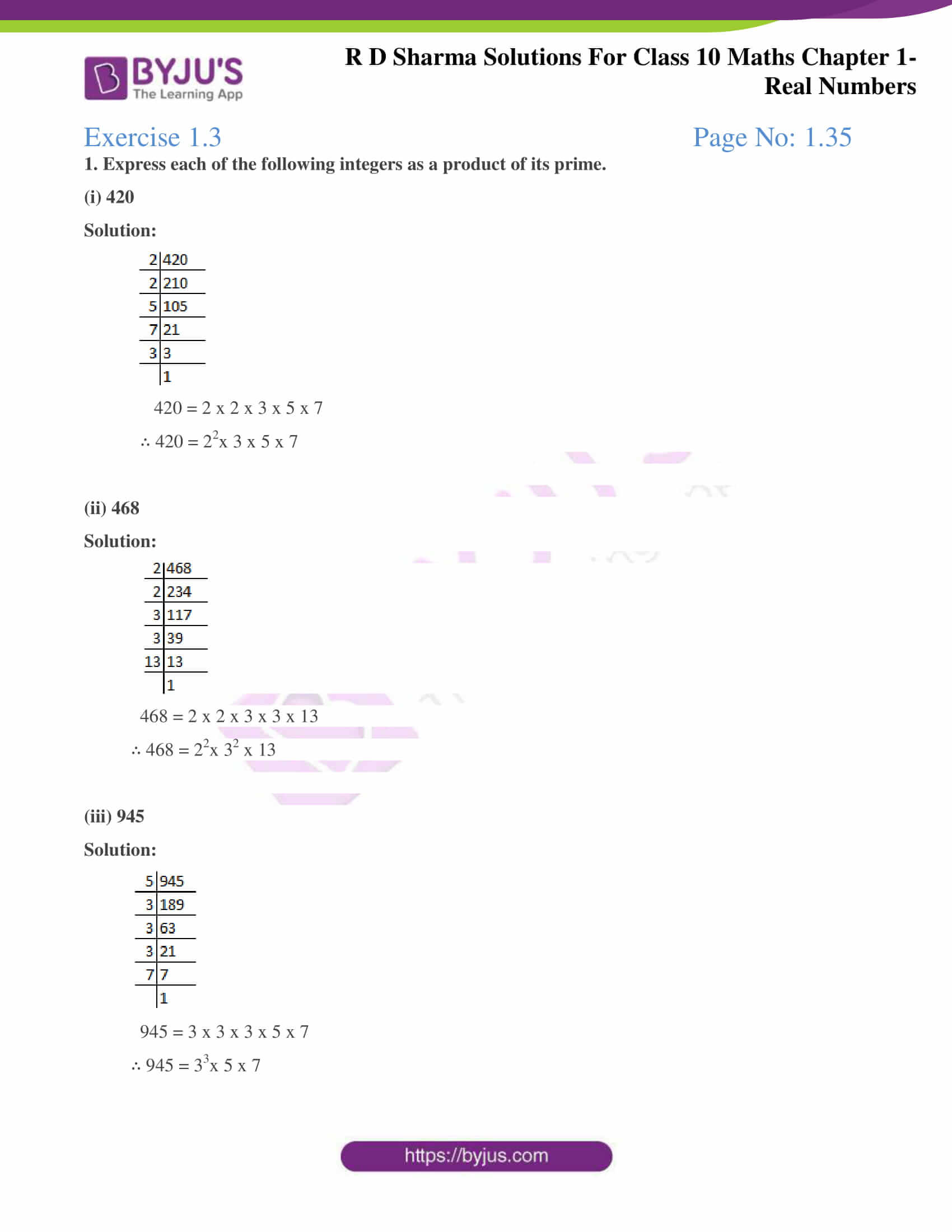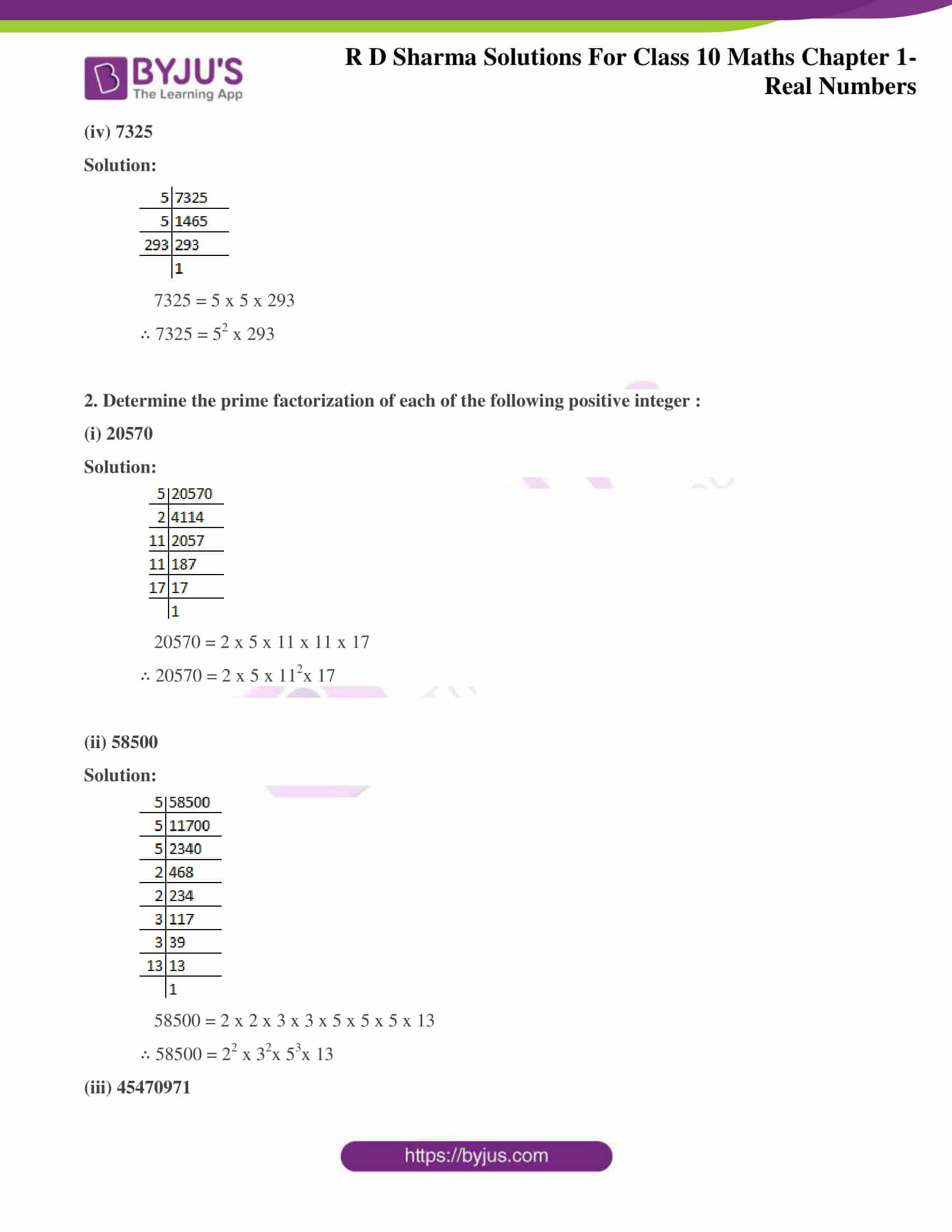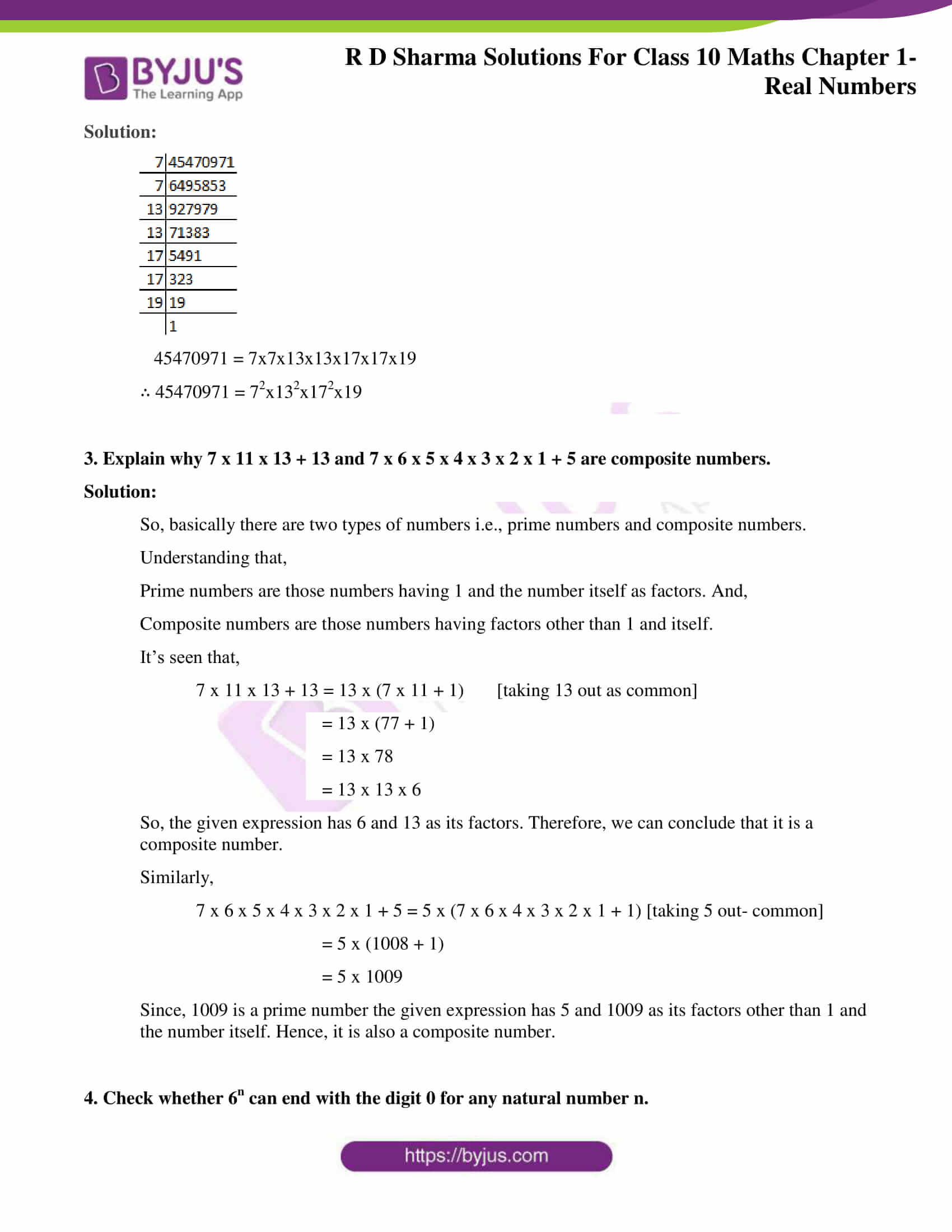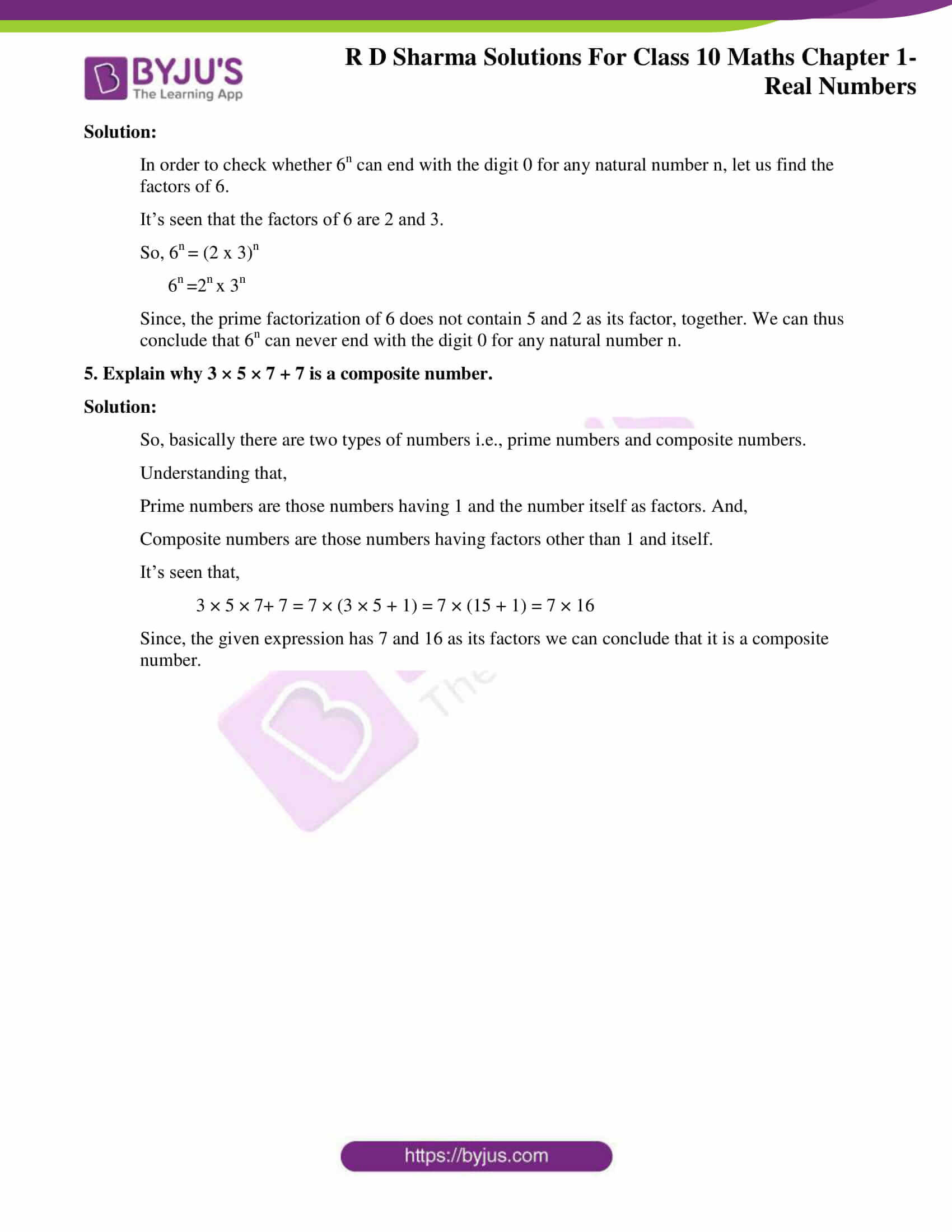### Access answers to Maths RD Sharma Solutions for Class 10 Chapter 1 Real Numbers Exercise 1.3

1. Express each of the following integers as a product of its prime.

(i) 420

Solution: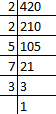420 = 2 x 2 x 3 x 5 x 7

∴ 420 = 22x 3 x 5 x 7

(ii) 468

Solution: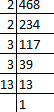468 = 2 x 2 x 3 x 3 x 13

∴ 468 = 22x 32 x 13

(iii) 945

Solution: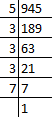945 = 3 x 3 x 3 x 5 x 7

∴ 945 = 33x 5 x 7

(iv) 7325

Solution: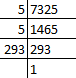7325 = 5 x 5 x 293

∴ 7325 = 52 x 293

2. Determine the prime factorization of each of the following positive integer :

(i) 20570

Solution: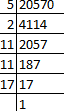20570 = 2 x 5 x 11 x 11 x 17

∴ 20570 = 2 x 5 x 112x 17

(ii) 58500

Solution: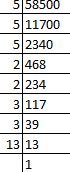58500 = 2 x 2 x 3 x 3 x 5 x 5 x 5 x 13

∴ 58500 = 22 x 32x 53x 13

(iii) 45470971

Solution: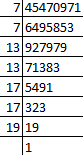45470971 = 7x7x13x13x17x17x19

∴ 45470971 = 72x132x172x19

3. Explain why 7 x 11 x 13 + 13 and 7 x 6 x 5 x 4 x 3 x 2 x 1 + 5 are composite numbers.

Solution:

So, basically there are two types of numbers i.e., prime numbers and composite numbers.

Understanding that,

Prime numbers are those numbers having 1 and the number itself as factors. And,

Composite numbers are those numbers having factors other than 1 and itself.

It’s seen that,

7 x 11 x 13 + 13 = 13 x (7 x 11 + 1) [taking 13 out as common]

= 13 x (77 + 1)

= 13 x 78

= 13 x 13 x 6

So, the given expression has 6 and 13 as its factors. Therefore, we can conclude that it is a composite number.

Similarly,

7 x 6 x 5 x 4 x 3 x 2 x 1 + 5 = 5 x (7 x 6 x 4 x 3 x 2 x 1 + 1) [taking 5 out- common]

= 5 x (1008 + 1)

= 5 x 1009

Since, 1009 is a prime number the given expression has 5 and 1009 as its factors other than 1 and the number itself. Hence, it is also a composite number.

4. Check whether 6n can end with the digit 0 for any natural number n.

Solution:

In order to check whether 6n can end with the digit 0 for any natural number n, let us find the factors of 6.

It’s seen that the factors of 6 are 2 and 3.

So, 6n = (2 x 3)n

6n =2x 3n

Since, the prime factorization of 6 does not contain 5 and 2 as its factor, together. We can thus conclude that 6n can never end with the digit 0 for any natural number n.

5. Explain why 3 × 5 × 7 + 7 is a composite number.

Solution:

So, basically there are two types of numbers i.e., prime numbers and composite numbers.

Understanding that,

Prime numbers are those numbers having 1 and the number itself as factors. And,

Composite numbers are those numbers having factors other than 1 and itself.

It’s seen that,

3 × 5 × 7+ 7 = 7 × (3 × 5 + 1) = 7 × (15 + 1) = 7 × 16

Since, the given expression has 7 and 16 as its factors we can conclude that it is a composite number.